# Long exact sequence of homology of a triple

This article defines a long exact sequence of homology groups, for topological spaces or pairs of topological spaces

## Definition

Suppose$A \subset B \subset C$ are topological spaces (each with the subspace topology from the bigger one). The long exact sequence of homology of this triple (usually denoted as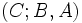$(C;B,A)$) is: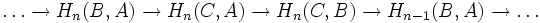$\ldots \to H_n(B,A) \to H_n(C,A) \to H_n(C,B) \to H_{n-1}(B,A) \to \ldots$

where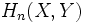$H_n(X,Y)$ denotes the relative homology.

## Particular cases

If$A$ is empty, we get the long exact sequence of homology of a pair, namely the pair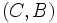$(C,B)$.

## For various homology theories

### For homologies arising from a chain complex

If the homology theory involves homology of a chain complex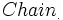$Chain_.$, then the above can be interpreted as the long exact sequence of homology arising from the following short exact sequence of relative chain complexes:$0 \to Chain_.(B,A) \to Chain_.(C,A) \to Chain_.(C,B) \to 0$

In particular, this description works for singular homology, cellular homology and simplicial homology.